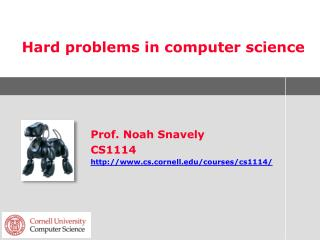# Hard problems in computer science - PowerPoint PPT PresentationDownload PresentationHard problems in computer science

Hard problems in computer science
Download Presentation## Hard problems in computer science

- - - - - - - - - - - - - - - - - - - - - - - - - - - E N D - - - - - - - - - - - - - - - - - - - - - - - - - - -
##### Presentation Transcript

1. Hard problems in computer science Prof. Noah Snavely CS1114 http://www.cs.cornell.edu/courses/cs1114/

2. Administrivia • Assignments: • A6 due Friday • Prelim 3 next Thursday • Review in class Tuesday • Final project proposals • Most of you now have feedback • Demo session on Tuesday, May 15, 1:30-3:30

3. Puzzle • Does this program terminate? i = 0; while true i = i + 1; end

4. Puzzle • How about this one? c= 2; while true for a = 2:c for b = 2:c if a^3 + b^3 == c^3 return; end end end c = c + 1; end

5. Hard problems in computer science • Many problems in computer science are “polynomial time” problems • We know of an algorithm that solves the problem exactly in O(nk) time, for some constant k • Examples? • Many other problems have no known polynomial time algorithm • E.g., problems whose fastest known algorithm takes O(2n) time [exponential time]

6. Hard problems in computer science • Other problems cannot be solved at all in general • Given a program written in Matlab, does that program ever terminate? • A version of the halting problem • Vaguely related to the puzzle a moment ago

7. Exponential time algorithms • Many other problems have no known polynomial time algorithm • E.g., algorithms whose best solution takes O(2n) time • If it takes 1 second to solve such a problem with n = 100 • Then it takes 2 seconds to solve for n = 101 • And it takes 250 seconds to solve for n = 150 • About 3.6 million years

8. Travelling Salesman Problem (TSP) • Classic hard problem in CS • Problem statement: • Given a weighted, complete graph with n nodes • Compute a tour that starts and ends at the same nodes, and visits all other nodes • Find such a tour that has the lowest total cost • A tour is also just a permutation of the nodes

9. Subway challenge

10. Solving the TSP • What kind of algorithm might solve the TSP? • Can you come up with an example that breaks your algorithm?

11. Solving the TSP • What if the graph is metric? • Means that nodes could be laid out on the plane, with weights corresponding to distance in the plane • Easy to find a good approximation algorithm (on board)

12. Other hard problems in CS • Hamiltonian cycle • Does a given graph contain a Hamiltonian cycle? • (Very related to TSP) • Graph coloring • Can a given graph be colored with k colors?

13. Other hard problems in CS • Sudoku on n2 x n2 boards of n x n blocks • General algorithm for solving Sudoku?